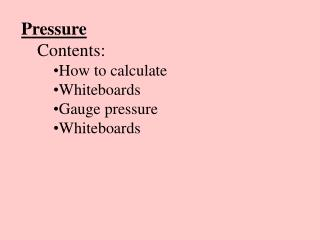DownloadDownload PresentationPressure Contents: How to calculate Whiteboards Gauge pressure Whiteboards

# Pressure Contents: How to calculate Whiteboards Gauge pressure Whiteboards

Télécharger la présentation## Pressure Contents: How to calculate Whiteboards Gauge pressure Whiteboards

- - - - - - - - - - - - - - - - - - - - - - - - - - - E N D - - - - - - - - - - - - - - - - - - - - - - - - - - -
##### Presentation Transcript

1. Pressure • Contents: • How to calculate • Whiteboards • Gauge pressure • Whiteboards

2. Pressure is in Force per unit area • P = F/A • F = Force in N • A = Area in m2 • P = pressure in N/m2 (Pascals - Pa) • Demo - 1 m2 • Example - a 2.4 kg box measures 15 cm by 25 cm on the base. What is the pressure under the box? • F = mg • A = LxW TOC

3. Pressure 1 | 2 | 3 TOC

4. What is the pressure of 42 N on a 20. cm x 32 cm plate? A = (.20 m)(.32 m) = .064 m2 P = F/A = (42 N)/(.064 m2) W 660 Pa

5. What force does 3200 Pa exert on a 78.0 cm x 182 cm pane of glass? A = LxW A = (.780 m)(1.82 m) = 1.4196 m2 P = F/A, F = PA = (3200 Pa)(1.4196 m2) W 4540 N

6. A hydraulic jack lifts a 31360 N car using a pressure of 1.38 MPa (M = x 106) What is the diameter of the cylinder? Find area A = r2 for a circle Diameter = 2r P = F/A, A = F/P = (31360 N)/(1.38 x 106 Pa) A = .022725 m2 A = r2 r = .08505 m d = 2r W .170 m

7. Gauge pressure Most pressure gauges compare to Atmospheric (i.e. this room is at 0 Gauge) Absolute p is 1 atm more P = Pgauge + 1 atm 1 atm = 1.013 x 105 Pa = 101.3 kPa = 760 Torr = 14.7 psi Demo - Magdeberg Hemispheres Example – What is the absolute pressure (In Torr and Pa) if you have a gauge pressure of 312 Torr? 312 + 760 = 1072 Torr absolute (1.013 x105 Pa/atm)(1072 Torr)/(760 Torr/atm) = 1.43E5 Pa TOC

8. Gauge Pressure 1 | 2 TOC

9. What is the absolute pressure if you read 35 psi gauge? Answer in psi (1 atm = 14.7 psi) and Pascals P = Pgauge + 1 atm P = 35 psi + 14.7 psi = 49.7 psi 1.013E5*49.7/14.7 = 3.42E5 Pa W 49.7 psi, 3.42E5 Pa

10. If you have an absolute pressure of 812 Torr, what is the gauge pressure? Answer in Torr (1 atm = 760 Torr) P = Pgauge + 1 atm 812 = Pgauge + 760 Torr Pgauge = 52 Torr W 52 Torr

11. What is the absolute pressure if the gauge pressure is 2.17 x 105 Pa (1 atm = 1.013 x 105 Pa = 101.3 kPa) P = Pgauge + 1 atm P = 2.17 x 105 Pa + 1.013 x 105 Pa W 3.18 x 105 Pa

12. If you have an absolute pressure of 42.0 kPa, what is the gauge pressure in kPa? (1 atm = 1.013 x 105 Pa = 101.3 kPa) P = Pgauge + 1 atm 42.0 kPa = pgauge + 101.3 kPa W -59.3 kPa